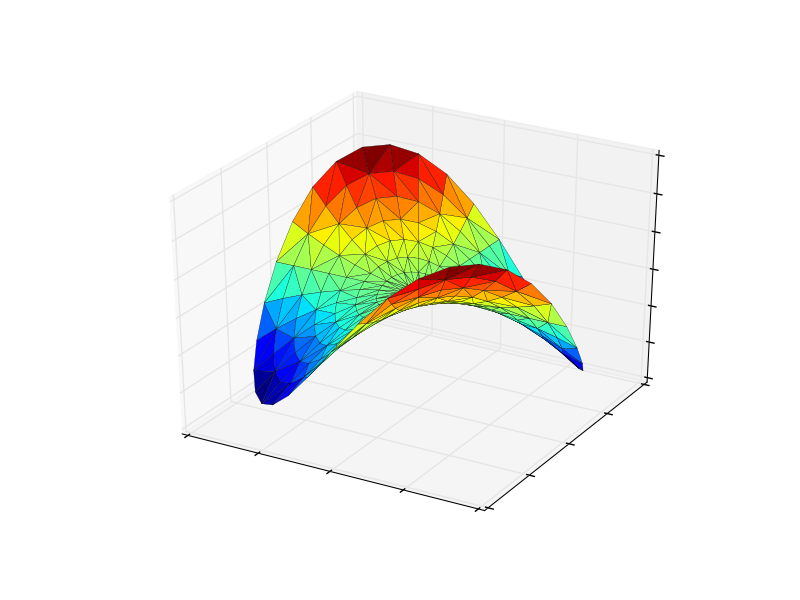# subtle change in trisurf image results

The mplot3d tests are not run automatically, so I have no idea when this change happened. But I would suspect it is related to the changes in triangulation rather than with mplot3d itself. I have zero expertise to say if one result is more correct than the other. See attached.

I should note that this particular difference was within the default tolerance. It was the rendering to PDF that seemed to have enough difference to trigger a failure.

Ben RootBen,

You are right, this change occurred when the underlying triangulation code
was switched to using qhull. Both before and after pictures are equally
correct.

A rectangle in the x-y plane is split into two triangles by adding a
diagonal. The shortest of the two diagonals should added, so for the
analytical solution either diagonal is equally valid. In practice the
choice of diagonal depends on the order of operations in the underlying
algorithm and how these interact with finite machine precision.

Rather than just changing the test image, a better solution would be to
modify the relevant example/test code to avoid such degenerate cases that
can give problems in the future. The source code for trisurf3d_demo (
http://matplotlib.org/examples/mplot3d/trisurf3d_demo.html) is clearly
derived from tripcolor_demo (
http://matplotlib.org/examples/pylab_examples/tripcolor_demo.html), but
omits the key line near the beginning of

angles[:,1::2] += math.pi/n_angles

Putting this line back in will avoid similar problems in the future, and
improve the images too.

Ian

···

On 25 June 2014 17:55, Benjamin Root <ben.root@...553...> wrote:

The mplot3d tests are not run automatically, so I have no idea when this
change happened. But I would suspect it is related to the changes in
triangulation rather than with mplot3d itself. I have zero expertise to say
if one result is more correct than the other. See attached.

I should note that this particular difference was within the default
tolerance. It was the rendering to PDF that seemed to have enough
difference to trigger a failure.